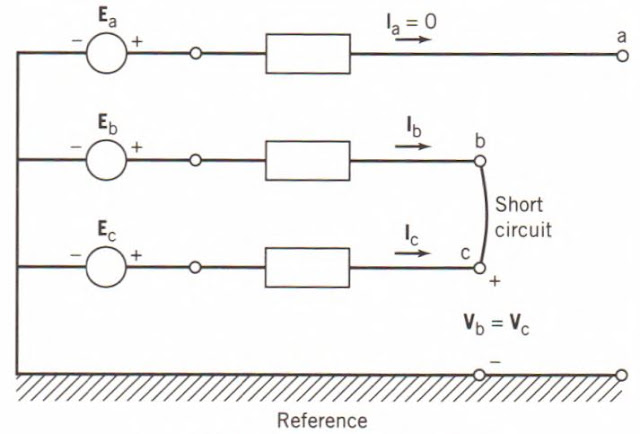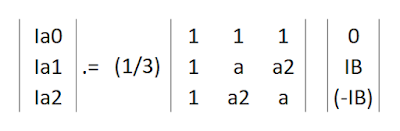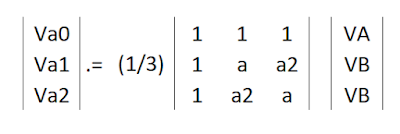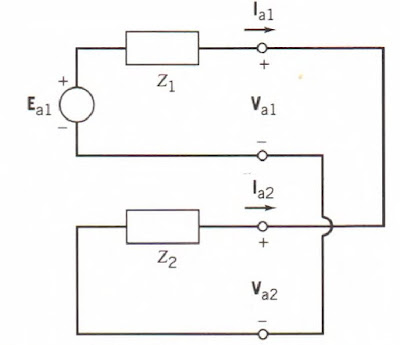Line to Line Fault

An unbalanced line-to-line fault in an electrical system refers to a type of short-circuit that occurs between two phases of a three-phase system, resulting in an imbalance in the current flowing through the system. This can occur when a conductor comes into contact with another conductor or when a conductor comes into contact with the ground. Unbalanced line-to-line faults can be caused by a variety of factors including equipment failure, human error, and natural disasters.

An unbalanced line-to-line fault can cause significant damage to the electrical system, including equipment failure and power outages. It can also lead to dangerous conditions for personnel working on or near the electrical system.

As mentioned above, this type of fault occurs when two phases of the three-phase line accidentally connected each other. In this case, the fault current will flow for both phases involved. From the given diagram, the fault occurs in phases B and C while phase A remain unfaulted.

From this scenario, the system parameters can be considered as follows:

• Fault current at phase A = 0 (since no fault current is flowing in phase A)
• Fault current at phase B = If-A
• Fault current at phase C = If-C.
• Here we can also see that voltages at phase B and phase C will be equal during fault, thus VB = VC.

Using sequence network matrix formula, we can plot the given values as follows.Symmetrical Components Matrix Formula in Line to Line Fault
From this matrix equation, we can get a value of,
• Ia0 = 0
• Ia1 = - Ia2
Which means, the zero sequence current in a Line to Line fault is not involved while the positive sequence current and negative sequence current is opposing each other.

In the sames sense, the matrix formula can also gives us the value of the voltages,Symmetrical Components Matrix Formula of Line to Line Fault

Note that in this case, VB = VC. By manipulating equations we can get Va1 = Va2.

From the result of two matrix equations involving current and voltage, we can develop a sequence network which describes as that the positive and negative sequence is in parallel with each other.Equivalent Sequence Network for Line to Line Fault

This figure satisfies the equation Va1 = Va2 and Ia1 = (-Ia2).

For sample calculation, see the following article Example: Line to Line Fault Calculation

In conclusion, unbalanced line-to-line faults in electrical systems are a serious concern as they can cause significant damage to equipment and lead to power outages. These types of faults can be caused by a variety of factors, including equipment failure, human error, and natural disasters. To detect and mitigate these faults, it is important for electrical systems to have proper protection and monitoring systems in place, such as Distance protection, over current protection, differential protection.

It is also important to have procedures in place for isolating the faulted section of the system and rerouting power to keep the rest of the system operational. With proper planning and maintenance, electrical systems can minimize the impact of unbalanced line-to-line faults and maintain the reliability of the power supply.